### Astrophysics

The Sun is an energy powerhouse. By our best measurements, the Sun emits energy at a rate of

$L_\odot=\SI{3.83e26}{\watt}.$

A Watt $(\si{\watt})$ is an abbreviation for $1$ Joule per second, so this is a tremendous amount of power. The fundamental astrophysical quantity $L_\odot$ is called the Sun's luminosity.

In this quiz, we are going to work out how the luminosity $L$ of any star is related to its surface temperature $T$. Then, we will construct the iconic Hertzsprung-Russel (HR) diagram, which captures many of the questions that astronomy was invented to address.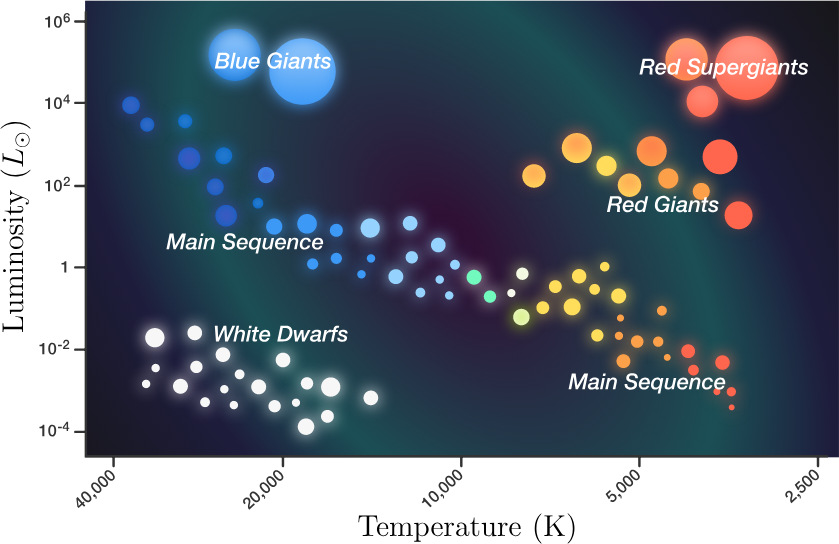# HR Diagram

Most of the radiation emitted by the Sun is in the form of photons. In the last quiz we saw that matter emits photons on a spectrum dependent on temperature, but how many photons are we talking about?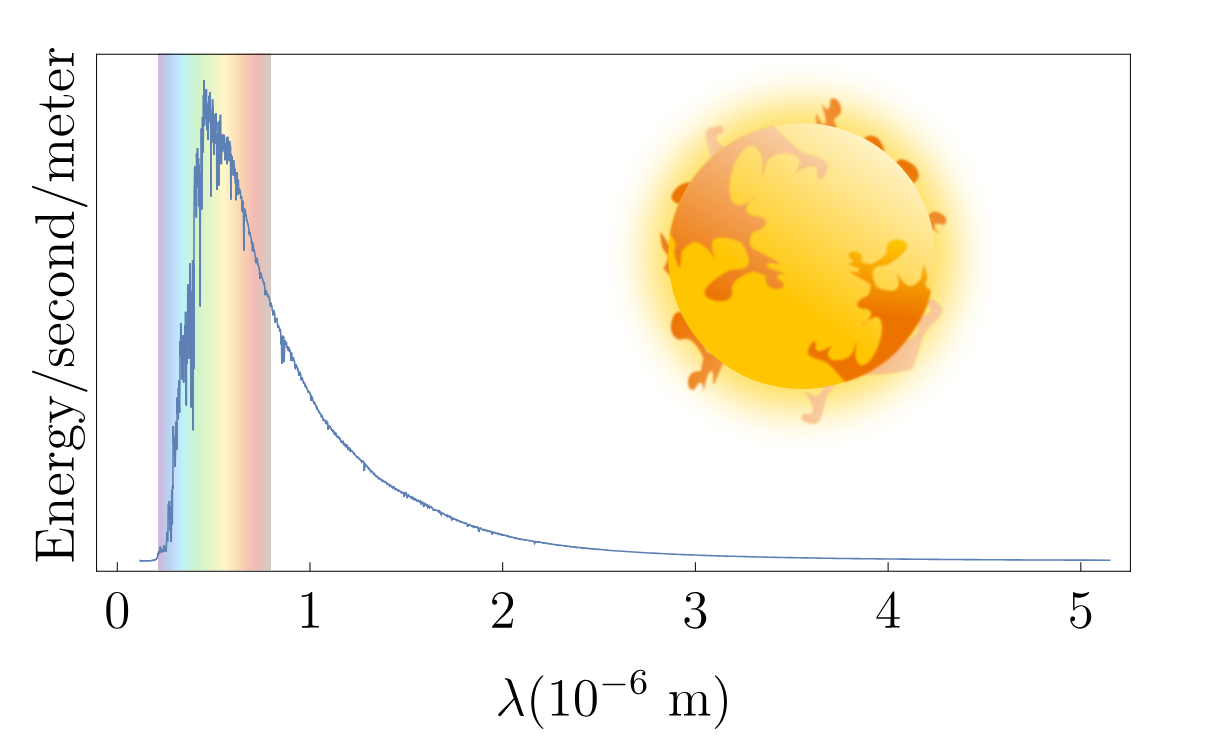For the purpose of estimation, suppose the Sun only emits photons with wavelength $\SI{500}{\nano\meter}.$ About how many photons are emitted each second?


Details and Assumptions:

• In the last quiz, using $E=hf,$ we calculated that a $\SI{500}{\nano\meter}$ photon has an energy of about $\SI{4e-19}{\joule}.$
• The luminosity of the Sun is $L_\odot=\SI{3.83e26}{\watt}.$

# HR Diagram

Because so many photons are escaping the Sun's surface each second, equal surface areas emit equal energy each second (as long as they are at the same temperature). As a result, the luminosity of a star depends on its temperature and its surface area.

An estimate of how much power is radiated by a star with temperature $T$ and area $A$ is contained in Stefan's law $L=\sigma A T^4,$ where $\sigma=\SI[mode=text]{5.67e-8}{W.m^{-2}.K^{-4}}.$

Which has a higher luminosity?

A. $\$ The Sun $($with a surface temperature of $\SI{5800}{\kelvin})$
B. $\ \,$A star with double the diameter of the Sun with the same surface temperature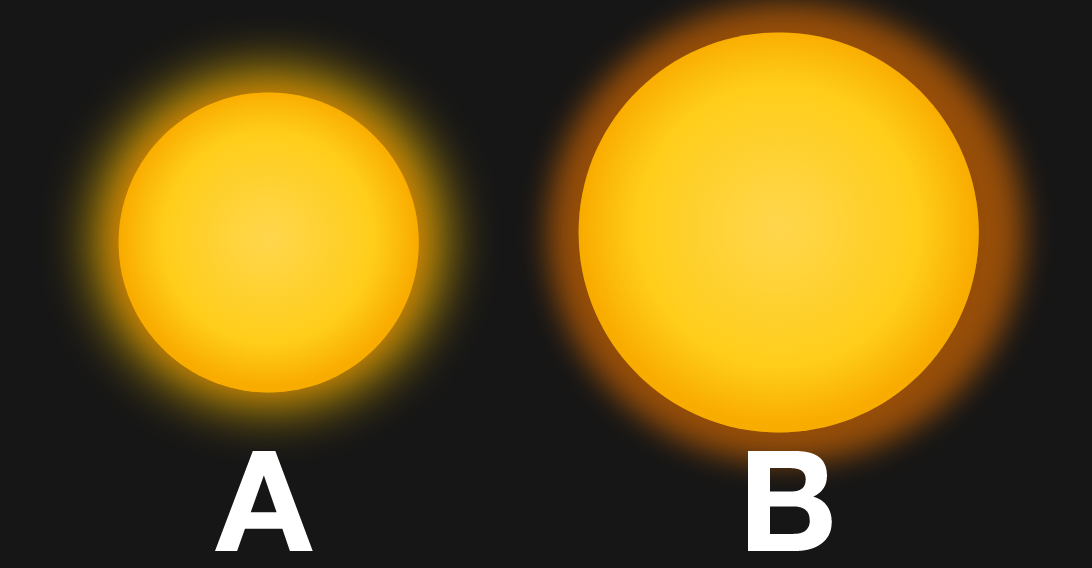# HR Diagram

In practice, we would be hard-pressed to observe a star with the Sun's surface temperature that is so much larger, as in the previous question. We will see in a later chapter that more massive stars have hotter cores, which can result in hotter surface temperatures as well.

Which star has a higher luminosity?

A.$\$ A reddish star
B.$\$ A bluish star with double the diameter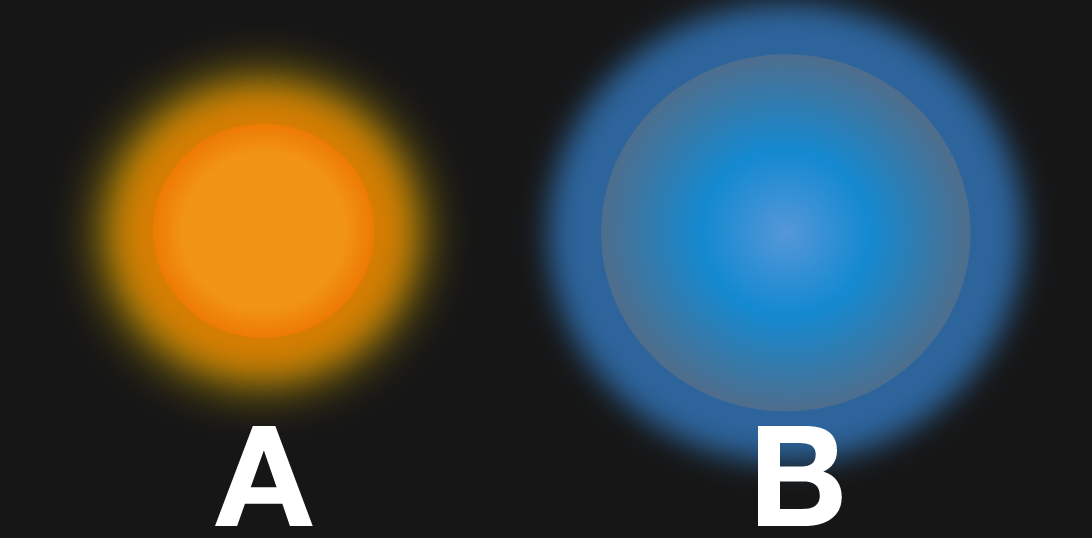Details and Assumptions:

• A typical wavelength of reddish light is $\SI{700}{\nano\meter}$ and a typical wavelength of bluish light is $\SI{400}{\nano\meter}.$
• Recall Wien's displacement law: $\lambda_\text{max} T = \SI{0.0029}{\meter\cdot\kelvin}.$

# HR Diagram

For a nearby star, two easy-to-measure parameters are readily extracted from its emission spectrum: peak wavelength (the wavelength at which most radiation is emitted) and luminosity. (In the next chapter, we will explore exactly how luminosity is obtained from measurement of a star's spectrum.)

In the early 20$^\text{th}$ century Ejnar Hertzsprung and Henry Norris Russel extracted these data from the spectra of many stars in the Pleiades Cluster. Expecting a relationship between $L$ and $T$ based on the intuition of the previous question, they decided to plot luminosity against peak wavelength and noticed that most of the stars fell on roughly a line.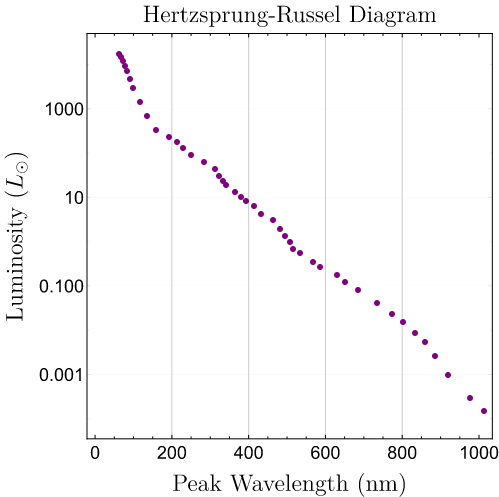Note that the luminosity axis is logarithmic and scaled by the luminosity of the Sun.

Which stars in the diagram have the highest surface temperature?

# HR Diagram

Using Wien's displacement law, astronomers have plotted the luminosity of thousands of stars against their surface temperatures. They discovered that most stars fall on a line of stars running from the top left to bottom right of the diagram—so-called main sequence stars, including our Sun (labeled A).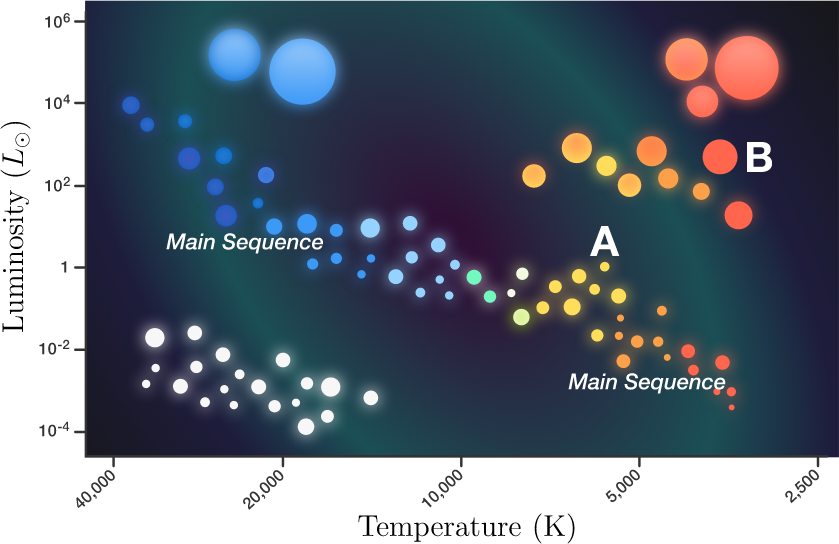Note that the luminosity axis is scaled by the luminosity of the Sun.

However, some stars do not fall on the main sequence. For example, star B has very different properties. Use Stefan's law to calculate its radius.


Details and Assumptions:

• Stefan's law is $L = \sigma A T^4,$ where $\sigma=\SI[mode=text]{5.67e-8}{W.m^{-2}.K^{-4}}.$
• Star B has a luminosity of $\SI{7.7e29}{\watt}$ ($2000$ times greater than the Sun's) and a surface temperature of $\SI{3000}{\kelvin}.$
• Assume Star B is spherical with surface area $A=4\pi r^2.$

# HR Diagram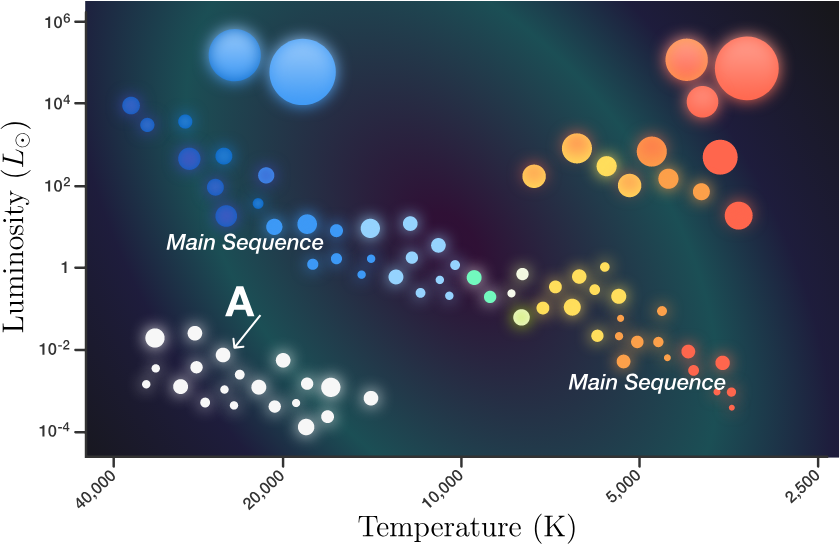Another distinct group of stars sits away from the main sequence on the bottom left part of the HR diagram. Estimate the radius of the star labeled A in this group.

Details & Assumptions

• Estimate the luminosity and temperature from the HR diagram. Note that the luminosities on the plot are displayed as a multiple of the Sun's luminosity: $L_\odot=\SI{3.83e26}{\watt}.$

# HR Diagram

When Hertzprung and Russell first came up with their famous diagram, it was not known what caused stars to fall into one of the three regions identified—red giants, white dwarfs, or main sequence. Why should only certain combinations of temperature and luminosity be allowed?

Over the following years, astronomers noticed that star clusters containing many hot, bright, main sequence stars had few red giant and white dwarf stars. Conversely, clusters with many red giants and white dwarfs had few hot main sequence stars. They hypothesized that stars evolve on the HR diagram, changing from one type to another as a cluster ages.

In the stars chapter, you will discover the processes that can turn one star into another with dramatically different properties.

×